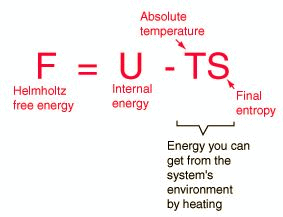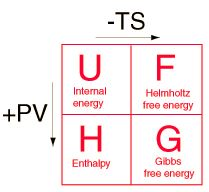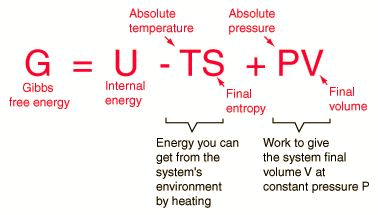# Helmholtz Free Energy and Gibbs Free Energy - CSIR-NET Physical Sciences Physics Notes | EduRev

## Physics : Helmholtz Free Energy and Gibbs Free Energy - CSIR-NET Physical Sciences Physics Notes | EduRev

The document Helmholtz Free Energy and Gibbs Free Energy - CSIR-NET Physical Sciences Physics Notes | EduRev is a part of the Physics Course Physics for IIT JAM, UGC - NET, CSIR NET.
All you need of Physics at this link: Physics

Helmholtz Free Energy

Four quantities called "thermodynamic potentials" are useful in the chemical thermodynamics of reactions and non-cyclic processes. They are internal energy, the enthalpy, the Helmholtz free energy and the Gibbs free energy. The Helmholtz free energy F is defined byThe internal energy U might be thought of as the energy required to create a system in the absence of changes in temperature or volume. But if the system is created in an environment of temperature T, then some of the energy can be obtained by spontaneous heat transfer from the environment to the system. The amount of this spontaneous energy transfer is TS where S is the final entropy of the system. In that case, you don't have to put in as much energy. Note that if a more disordered (higher entropy) final state is created, less work is required to create the system. The Helmholtz free energy is then a measure of the amount of energy you have to put in to create a system once the spontaneous energy transfer to the sytem from the environment is accounted for.

The four thermodynamic potentials are related by offsets of the "energy from the environment" term TS and the "expansion work" term PV. A mnemonic diagram suggested by Schroeder can help you keep track of the relationships between the four thermodynamic potentials.Gibbs Free Energy

Four quantities called "thermodynamic potentials" are useful in the chemical thermodynamics of reactions and non-cyclic processes. They are internal energy, the enthalpy, the Helmholtz free energy and the Gibbs free energy. The Gibbs free energy G is defined byThe internal energy U might be thought of as the energy required to create a system in the absence of changes in temperature or volume. But as discussed in defining enthalpy, an additional amount of work PV must be done if the system is created from a very small volume in order to "create room" for the system. As discussed in defining the Helmholtz free energy, an environment at constant temperature T will contribute an amount TS to the system, reducing the overall investment necessary for creating the system. This net energy contribution for a system created in environment temperature T from a negligible initial volume is the Gibbs free energy.

The change in Gibbs free energy, ΔG, in a reaction is a very useful parameter. It can be thought of as the maximum amount of work obtainable from a reaction. For example, in the oxidation of glucose, the change in Gibbs free energy is ΔG = 686 kcal = 2870 kJ. This reaction is the main energy reaction in living cells.

Offer running on EduRev: Apply code STAYHOME200 to get INR 200 off on our premium plan EduRev Infinity!

159 docs

,

,

,

,

,

,

,

,

,

,

,

,

,

,

,

,

,

,

,

,

,

;